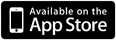# iPhone ABTC Basic CalculatorArtikel wurde nicht gefunden

ABTC Basic Calculator is designed to help young math students visualize and understand the process behind basic mathematical functions even when they’re using a calculator. It’s a smart way to reinforce addition, subtraction, multiplication and division principles in new math students, and keep the concepts fresh in the minds of middle school level learners.

How exactly does it do this, you may ask? By breaking down equations line by line. For example, when users input a basic addition problem into the app’s standard calculator style input, it will mimic the way this equation would appear if it was being worked on pencil and paper, complete with carry overs and extended decimals.

I’ll be honest, math was never my best topic as a student. That may or may not be the fault of my scientific calculator, since it did most of my math for me after a certain point, making it much easier to forget the basics. Addition, subtraction, multiplication and division are the blocks many higher math theories are built on. So the idea behind ABTC Basic Calculator really appeals to me.

The basic driving principle is that explaining a process in a visual way can facilitate a deeper, clearer way of learning. This app does have some limitations. You can’t enter a set of parenthesis, but it does help delineate standard mathematical order of operations in line functions. And I wish the app had more plain English explanations, or help files for certain types of problems.

Also, it’s not the most visually appealing app I’ve ever seen. There aren’t any fun characters or splashy animations to help make it easier for children to swallow the math pill. But it does function beautifully, and present math in a cool, new way.

Bottom line

ABTC Basic Calculator is a great way to support learning basic math principles and has an awesome price tag. It could use a bit of a facelift.

ABTC Basic Calculator is a four-function calculator that displays the steps a person would makes with a pen and paper for problems in addition, subtraction, multiplication, long division, and the correct order of solving equations with multiple operations. This preserves the speed and accuracy that traditional calculator have, and helps the user learn the proccess through repetition. If you would like to view a demo of the ABTC Basic Calculator, please visit youtube and search for iPhone ABTC Basic Calculator, or go to the link below.

http://www.youtube.com/watch?v=oY5BVzuYZSw. Regular Price: .99